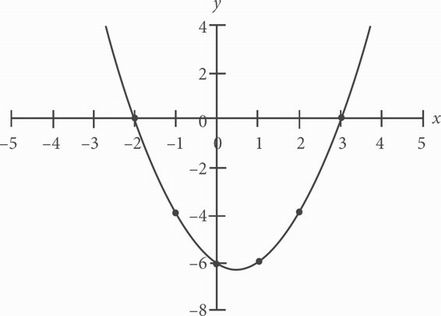# SAT Math Multiple Choice Question 227: Answer and Explanation

### Test Information

Question: 227

2.Which of the following quadratic equations is represented in the graph above?

• A. y = x2 - x - 6
• B. y = x2 + x - 12
• C. y = x2 + 6x + 9
• D. y = x2 + 5x + 6